# Modelling of the Effect of Basic Macroeconomic Indecencies in the National Budget Deficit in Ukraine

Nataliia Savchuk, PhD in economics, Associate Professor, International Finance Dpt., Vagym Getman Kyiv National Economic University.

### Summary

The multiple linear regression model is applied, by approximation and smoothing, to the time series of the key macroeconomic indicators of the Ukraine. The calculated regression statistics and the conducted ANOVA are presented according to the remaining confidence, which is set at 0.97. The most significant impact on the state budget reveals a second variable: the ratio of trade to the balance of payments, following which the impact on deficit depends on taxes. GDP and public debt do not significantly affect the state budget deficit.

Significance of the problem. One of the important tasks of our days is to develop an economic and mathematical model to show the influence of the key macroeconomic indicators of the Ukraine, such as GDP, balance of trade, balance of payment, government debt and taxes on the Ukraine’s budget deficit.

Statement of the problem. In an analysis of the basic characteristics of the Ukrainian budget, we presumed a functional dependence of the deficit/surplus of the Ukraine’s budget on key macroeconomic indicators such as GDP, balance of trade, balance of payment, government debt and taxes.

Table 1 shows the selected key macroeconomic indicators that affect the budget deficit.

Table 1: Key macroeconomic indicators affecting budget deficit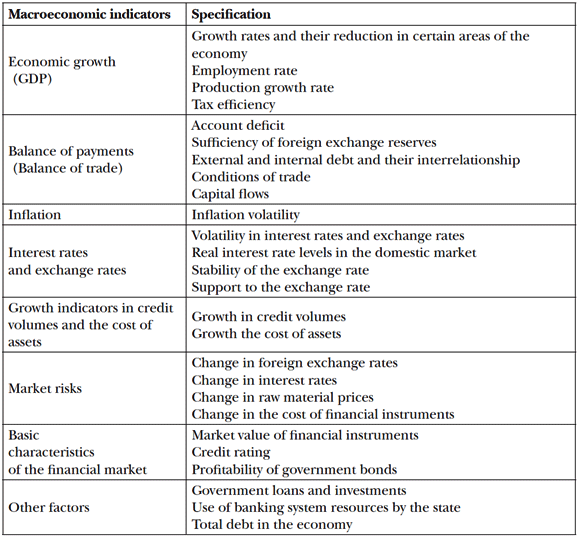This functional relationship can be represented as a model of linear multiple regression, with a target function of deficit/surplus in the Ukrainian budget, and is calculated with an input of independent variables (the Ukraine’s key macroeconomic indicators).

Analysisofstudiesandpublicationsdedicatedtotheproblem.Contemporary economic literature presents various models for influence the Ukraine’s key macroeconomic indicators have on the budget balance. In general, these are econometric single- and multi-factor linear regression models that describe the impact of budget deficit or of taxes on GDP or the influence of tax revenues on the country’s budget expenses etc.

Article 1 presents the model of interrelationship between the Ukraine’s budget deficit and indicators of macroeconomic dynamics, as illustrated by the model of the influence of taxes on the value of the Ukraine’s budget in the form of a two-factor regression model.1

Article 2 presents a model for the management of budget deficit and its components through the study of their growth rates, and an inverse relationship is revealed between the relative and absolute indicators of the budget deficit.2

Article 3 presents a regression model of dependence of GDP on the revenue of the Ukraine’s budget and retail sales. This model is used for making forecasts on GDP.3

Article 4 presents a linear model of forecast of the government debt, and smoothing was performed using the moving-average method on the basis of auto regression.4 Work 5 presents a linear regression model of the external direct and guaranteed debt, which depends on deficit of Ukraine’s budget. It is shown that reduction of the budget deficit results in increase of the budget.5 Work 6 shows fuzzy modelling of post-crisis economic processes with forecasting of the GDP. In our opinion, the drawback of such modelling is the insufficient statistical data required for implementation of this model. It is necessary, for example, to increase the amount of data by using quarterly data.6

V. V. Vitlinskyi’s studies7 analyse the importance of an optimal compromise between the country’s desire to reduce the budget deficit and the negative effect of increasing corporate taxes. It is emphasized that if the economy grows, taxes can be increased and, vice versa, if the economy recedes, high tax rates only enhance recession.

The study of the functional relation in the form of a linear multiple regression model is also interesting. In this model, the target function of deficit/surplus of the Ukraine’s budget depends on the key macroeconomic indicators.

Objective of the study:

• study the dynamics of the key macroeconomic indicators of the Ukraine;
• build a model for the impacts of the key macroeconomic indicators of Ukraine on the budget deficit;

Setting forth the basic assumptions. The statistical data of the presented independent variables of the key macroeconomic indicators (GDP, balance of trade, balance of payments, government debt and taxes) for the period between 1998 and 2013 must be considered during the same intervals of time and place of operation. In order to formalize data, we use the mathematical method of natural normalization, which does not change the economic indicator, i.e. the normalized (non-dimensional) data only comprise qualitative information.

By natural normalization, all the studied statistical data of the balance of Ukraine’s budget given in million hryvnias for the period between 1998 and 2013 become nondimensional within the range 0-1 after re-calculation with the following formula:8where:

i – data change index,

P*i – statistical data of the macroeconomic indicator,

miniP*i – minimum value in the statistical data,

maxiP*i – minimum value in the statistical data,

P*ir – fixed value in the statistical data,

Pr – recalculated non-dimensional value which corresponds to the fixed value of the statistical data.

The Pr values have no dimensions (millions hryvnias) and fall within the range of values.

From among the basic numerical approximation methods of non-dimensional time series points, we have chosen the approximation method for an analytical function, and for the table function of the Ukraine’s budget deficit for the period between 1998 and 2013, we have applied approximation by polynomials in the non-dimensional form.

Figure1 shows a polynomial approximation of points in the Ukraine’s budget deficit for the period between 1998 and 2013 in a non-dimensional form, using a polynomial of 6th degree (broken line in Figure1):

y = 0.00001x6 0.00007x5 0.142x4 0.1352x3–0.6398x2 1.3688x (2)

with the standard error of deviation being 0.829.

The forecast value is calculated by this approximation. The value is shown in Figure1for the year 2014 (see the continuation of the broken line beyond the bottom line of the points), and the forecast trend is a significant decrease.

Figure 1: Non-dimensional budget balance and approximation by a polynomial function of the 6th degree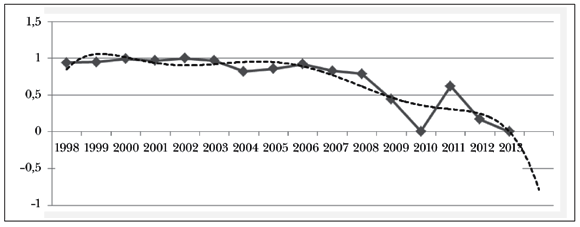Figure 2 shows the data smoothed by approximation of time series of non-dimensional budget deficit and the ratio of the balance of trade to the balance of payments for the period between 1998 and 2013.

Figure 2: Smoothed time series in the budget deficit and the ratio of the balance of trade to the balance of payments for the period between 1998 and 2013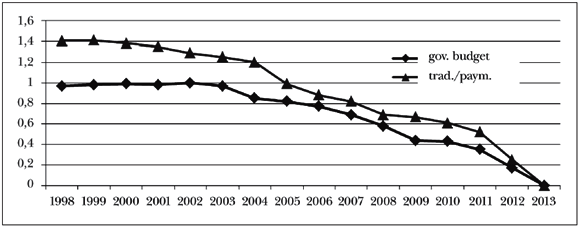Figure 2 shows that these two indicators have almost the same trend, which gives room for a hypothesis that the ratio of the balance of trade to the balance of payment on the budget deficit may have the most significant influence.

Figure3 shows the smoothed data of time series for non-dimensional variables like GDP, general government debt and taxes from the item of the budget for the period between 1998 and 2013. By analysing Figure3, it can be concluded that in 2010 the time series were almost identical and similar curves were drawn for the total government debt and taxes.

Figure 3: Smoothed time series of the key non-dimensional macroeconomic indicators: GDP, total debt and taxes, 1998–2013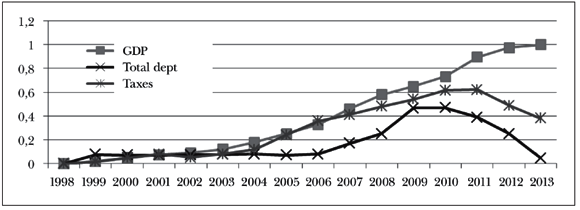The approximation and smoothing of the time series of the key macroeconomic indicators of the Ukraine allow the economic and mathematical modelling by multiple linear regression.

In our opinion, the analysis of Figures1 and 2 suggests that our hypothesis about the greatest influence that the ratio of the balance of trade to the balance of payments has on the budget balance can only be be confirmed after application of the linear multiple regression model.

If we consider the following dependences of the linear multiple regression:

y a0 a1x1 a2x2 a3x3 a4x4, (1)

where the target function Y is the country’s budget deficit which depends on the independent variables: x1 (GDP), x2 (ratio of the balance of trade to the balance of payment), x3 (total government debt), x4 (taxes).

Thus, for 15 observations, the standard error is 0.032999, the multiple R is 0.999267, normalized R2 = 0.907226, R2 = 0.998535.

Table2 shows the basic regression statistics of the multiple linear regression model of functional dependence of the country’s budget deficit on the key macroeconomic indicators.

Table2: Basic regression statistics of the multiple linear regression model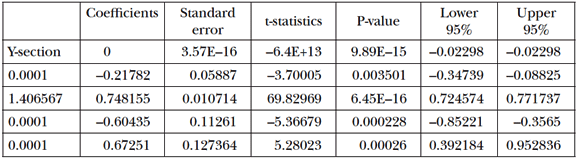Table2 shows that Fisher’s and Student’s test statistics, as well as the P-value fall within the appropriate range of alternative hypothesis acceptance.

Therefore, the equation of the multiple linear regression is as follows:

y = –0.21782x1 + 0.748155x2 – 0.60435x3 + 0.67251x4. (2)

It can be concluded that the greatest influence on the budget balance is made by the second variable – the ratio of the balance of trade to the balance of payments (coefficient 0.745185 > 0). It is followed by taxes, according to the degree of influence on the budget deficit (fourth variable with the coefficient of 0.672551 > 0). As far as GDP (coefficient –0.21782 < 0) and government debt (coefficient –0.21782 < 0) are concerned, they do not have a significant effect on the budget deficit.

Conclusions. In order to confirm the hypothesis about the availability of a functional dependence of deficit/surplus of the Ukraine’s budget on the key macroeconomic indicators such as GDP, balance of trade, balance of payment, government debt and taxes, we have done the following:

• The dynamics in recalculating the statistical series of the Ukraine’s budget deficit are shown for the period between 1998 and 2013 in million hryvnias. With the help of the natural normalization formula, the non-dimensional values of this dynamics in a non-dimensional form, which enables the presentation of the polynomial approximation of the points, using a polynomial of the 6th degree (1) with the standard error of deviation being 0.829, as a sufficient criterion of approximation. This allowed the calculation of the forecast value, and this forecast tends to decrease significantly.
• The dynamics of the key macroeconomic indicators (independent variable models), such as GDP, balance of trade, balance of payments, government debt and taxes of the Ukraine is analysed for the period between 1998 and 2013, and natural normalization is applied to it.
• A model of multiple linear regression was developed, which became possible after approximation and smoothing of the time series of the key macroeconomic indicators of the Ukraine. Regression statistics was calculated and an analysis of variance conducted. The remaining parts are also presented according to the confidence probability, given at 0.97. It is proved that the greatest influence is made by the second variable, i.e. the ratio of the balance of trade to the balance of payments. It is followed by taxes according to the degree of influence on the budget deficit. As far as GDP and government debt are concerned, they do not have a significant effect on the budget deficit.

#### Jegyzetek

• 1. K. Bazylevych – O. Tsaruk: Modeliuvannia vzayemozviazkiv defitsitu derzhavnoho biudzhetu Ukrainy z pokaznykamy makroekonomichnoyi dynamiky. www.tsaruk.com/docs/Bank2002_3.pdf
• 2. I. I. Diakonova: Biudzhetnyidefitsit i yoho rehuluvannia u perehidniy ekonomitsi Ukrainy 2000 r. Avtoref. dys. kand. ekon. nauk: 08.04.01. Ukr. akad. bank spravy, Sumy, 2000.
• 3. Y. M. Dryn – Y. S. Kovalskykh – Y. S. Ursaki: Ekonometrychna model zalezhnosti VVP vid dokhodiv derzhavnohobiudzhetu ta zahalnoho rozdribnoho tovaroobihu. perspektyva.dp.ua/files/conference/2014/01.31.01.2014-1.pdf
• 4. V. V. Barabanova: Zastosuvannia ekonomiko-matematychnykh modeley u prohnozuvanni derzhavnoho borhu. Efektyvna ekonomika, Dnipropetrovskyi derzhuniversytet. www.economy.nayka.com.ua/?op=1&z=1595
• 5. L. I. Bazhan – Y. N. Matveyeva: Analiz vzayemozviazku defitsytu derzhavnoho biudzhetu ta zovnishnioho derzhavnoho borhu. papers.univ.kiev.ua/vijskovo_specialni_nauky/articles/Analysis_of_interconnection_between_government_deficit_and_external_public_debt_18289.pdf
• 6. M. V. Sulym – L. I. Stefaniak: Vykorystannia ekspertnykh otsinok dlia nechitkoho modeliuvannia pislia kryzovykh ekonomichnykh protsessiv. www.lac.lviv.ua/fileadmin/www.lac.lviv.ua/data/pidrozdily/Naukovi_Vydannya/Vydan_Ekon/Docs/Visnyk_Ekonomika_Vypusk37.pdf
• 7. V. V. Vitlinskyi [ta in.]: Ekonomichnyiryzyki metody yoho vymiriuvannia: pidruchnyk dlia stud. ekon. spets. Instytut zmistu i metodiv navchannia, Kyivskyi derzh. ekonomichnyi universytet, [b.v.], K., 1996; V. V. Vitlinskyi – P. I. Verchenko: Analiz, modeliuvannia ta upravlinnia ekonomichnym ryzykom: navch.metod. posib. dlia samost. vyvchennia dystsypliny. Kyivskyi natsionalnyi ekonomichnyi un-t., KNEU, K., 2000; V. V. Vitlinskyi [ta in]: Matematychne programuvannia: navch.-metod. posib. dlia samost. vyvchennia dystsypliny. Kyivskyi natsionalnyi ekonomichnyi un-t., KNEU, K., 2001; V. V. Vitlinskyi: Modeliuvannia ekonomiky: navch. posibnyk.Kyivskyi natsionalnyi ekonomichnyi universytet, KNEU, K., 2003. - 406 s.: rys. - Bibliohr.: s. 403.; V. V. Vitlinskyi – H. I. Velykoivanenko: Ryzykolohia v ekonomitsi ta pidpryiemnytstvi. Kyivskyi natsionalnyi ekonomichnyi un-t., KNEU, K., 2004.
• 8. T. V. Bludova – I. A. Dzhaladova – O. I. Makarenko – H. V. Shuklin: Matematychna ekonomika. Navch. posib., KNEU, K., 2009.